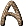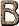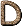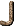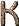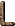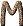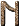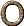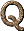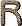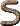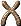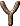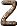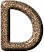d-Electron An orbital electron whose l quantum number is 2. D Stars Degenerate or White Dwarf Stars: Objects lying five or more magnitudes below the main sequence. Usually the following subgroups are distinguished: DC continuous spectrum, no lines clearly visible, DB only strong helium lines present, DA only strong hydrogen lines present, DO both He and H lines present, DZ no He nor H lines, but metallic lines present and DQ carbon lines (atomic or molecular) present. [JJ95] D Galaxy A supergiant radio galaxy (the most common type of radio galaxy) which has an elliptical nucleus surrounded by an extended envelope. Or, an optical galaxy with a very bright nucleus. In the Morgan classification, a galaxy with rotational symmetry but without pronounced spiral or elliptical structure (a dustless galaxy). In the Yerkes 1974 system a galaxy with an elliptical-like nucleus surrounded by an extensive envelope (see also R galaxy). [H76] D Layer The lowest part of Earth's ionosphere (starts at about 100 km). This is the layer that reflects "broadcast" radio waves. [H76] D Lines Two close spectral lines of neutral sodium (see Fraunhofer lines) at 5896 Ĺ (D1) and 5890 Ĺ (D2). [H76] D Ring The innermost ring of Saturn, discovered in 1969. [H76] Damped Oscillation An oscillation with an amplitude that progressively decreases with time. [DC99] Damping (a) In any oscillating system, a decrease in the amplitude of an oscillation due to the dissipation of energy. [H76] (b) The gradual decay of an oscillation or wave due to viscous drag forces. [Silk90] DARA Deutsche Agentur Fur Raumfahrt Angelegenheiten (Germany). [LLM96] Dark Current (a) The current that flows in a photoelectric cell when not illuminated. [H76] (b) Signals generated in a detector such as a CCD merely by the heat energy of the atoms which, through random jostling, results in the production of free electrons. Dark current is greatly reduced by physically cooling the detector. [McL97] Dark EnergyDark Halo (a) The massive outer region of the Milky Way that surrounds the disk and stellar halo. The dark halo consists mostly of dark matter, whose form is unknown. Though it emits almost no light, the dark halo outweighs the rest of the Galaxy. [C95] (b) Massive, nonluminous matter of unknown kind that surrounds and envelopes galaxies. [HH98] Dark Matter(a) Material astronomers cannot see but whose presence they believe in either because they detect its gravitational influence or because certain theories predict its existence. For example, astronomers believe that the outer part of the Galaxy harbors dark matter, because they notice its gravitational influence on the stars they can see; and inflationary cosmologists believe that the universe is full of dark matter, because inflation predicts that the universe has a large density. [C95] (b) Any form of matter which exists in the Universe in a non-luminous form. [C97] (c) Matter whose existence is inferred on the basis of dynamical studies - e.g., the orbits of stars, in galaxies - but which does not show up as bright objects such as stars and nebulae. Its composition is unknown: It might consist of subatomic particles, or of dim dwarf stars or black holes, or a combination of various sorts of objects. [F88] (d) Matter that is invisible to us because it emits little or no light. As much as 90%-99% of the mass of the universe may be dark. [HH98] (e) Matter that is detected only by its gravitational pull on visible matter. At least 90%, and possibly 99% of the matter in the universe is dark. The composition is unknown; it might consist of very low mass stars or supermassive black holes, but big-bang nucleosynthesis calculations limit the amount of such baryonic matter to a small fraction of the critical mass density. If the mass density is critical, as predicted by the simplest versions of inflation, then the bulk of the dark matter must be a gas of weakly interacting non-baryonic particles, sometimes called WIMPS (Weakly Interacting Massive Particles). Various extensions of the standard model of particle physics suggest specific candidates for the WIMPs. [G97] (f) Matter in the universe that we detect by its gravitational influences, yet do not see. Dark matter that has small random speed and is easily concentrated by gravity is called cold dark matter. Dark matter that has large random speed and is thus able to resist gravitational clumping is called hot dark matter. Recent models to explain the observed pattern of galaxy clustering can be characterized, in part, as to whether they invoke hot dark matter or cold dark matter. However, since we do not know what the dark matter is, we do not have any direct evidence of whether it is cold or hot. [LB90] Dark Nebula A relatively dense (up to 104 particles per cm3) cloud of interstellar matter whose dust particles obscure the light from stars beyond it and give the cloud the appearance of a region devoid of stars. [H76] Darwin Ellipsoids Ellipsoidal figures of equilibrium of homogeneous massive bodies describing circular orbits with a uniform angular velocity about each other on certain approximations relative to their mutual tidal influences. [H76] Darwinism Theory that species arise through the natural selection of random mutations that better fit changing conditions in a generally uniformitarian Earth. [F88] DAT Digital Audio Tape [LLM96] Data The outcome of a set of measurements from which inferences may be drawn, theories constructed, and so forth. [HH98] Data Model A description of the structure and organization of the data in a database. Specifically, the Data Model makes explicit the tables names, their contents, and how they are related to, and linked with, one another. The actual implementation of a data model is called the Database Schema. [BFM03] Data Rate The number of pieces of digital information being returned each second from an instrument. Measured in bits per second (bps) for serial information or bytes per second for parallel data. [McL97] Daughter Nuclide A given nuclide produced by radioactive decay from another nuclide (the parent). [DC99] Day An interval of 86400.51 seconds (see second, Systčme International), unless otherwise indicated. [S92] Day Numbers Quantities that facilitate hand calculations of the reduction of mean place to apparent place. Besselian day numbers depend solely on the Earth's position and motion; second-order day numbers, used in higher precision reductions, depend on the positions of both the Earth and the star. [S92] db Galaxy One of a small number of dumbbell-shaped radio galaxies. They might be called D systems with double nuclei, in which two elliptical nuclei share a common extended envelope. [H76] DC Direct Current Fixed voltages. For example, dc bias in CCD circuits. [McL97] Dead Reckoning Navigation by recording one's heading, velocity and elapsed time, with little or no reference to the stars. [F88] de Broglie Wavelength (Bar) The wavelength associated with a particle with a momentum p:bar = h/p. [H76] Debye Unit of electric-dipole moment, equal to that existing between a unit of positive charge and a unit of negative charge separated by a distance of 1 cm. 1 debye = 10-18 statcoulomb cm. [H76] Debye-Hückel Model The standard plasma model of an ionized classical gas. [H76] Debye Length A theoretical length which describes the maximum distance that a given electron can be from a given positive ion and still be influenced by the electric field of that ion in a plasma. Although according to Coulomb's law oppositely charged particles continue to attract each other at infinite distances, Debye showed that there is a cutoff of this force where there are other charged particles between. This critical separation decreases for increased density. lD = (kTe / 4nee2)1/2. [H76] Debye Unit A unit of electric dipole moment equal to 10-18 e.s.u. cm or 3.336 × 10-30 coulomb metre. The unit evolved naturally from the fact that many electric dipole moments for molecules are a multiple of 10-18 e.s.u. cm. Thus the dipole moment of hydrochloric acid is 1.05 × 10-18 e.s.u. cm; for aniline it is 1.5 × 10-18 e.s.u. cm. The unit first received a name in 1934, but it is frequently used in the abbreviated form, which is the letter D. It has also been defined as the product of the electronic charge and either the radius of the first Bohr orbit of hydrogen, giving a value of 2.54 × 10-18 CGS units, or one ĺngström, giving a value of 4.803 × 10-18 CGS units. It is named after P. J. W. Debye (1884-1966), the pioneer authority on polar molecules. The SI unit of electric dipole moment has not, as yet, received a name. [JM92] Decay The spontaneous disintegration of unstable (radioactive) nuclei to give other nuclei, accompanied by the emission of particles and/or photons. The product of a given decay may be stable or may itself be radioactive. In a sample of radioactive material the radioactivity falls with time; the activity is proportional to the amount of substance present. Decay follows an exponential law. At any time t the number (N) of original nuclei present is given by: N = N0 exp -t where N0 is the original number and y is a constant (the decay constant or disintegration constant) for a given nuclide. y is related to the half-life by: T = 0.693 15 y [DC99] Decay of the False Vacuum Since the universe is not inflating today, the inflationary theory depends on the fact that the false vacuum which drives inflation is metastable, so it will eventually decay. The theory requires that this decay happen after more than 100 doubling times of the exponential expansion, but it still happens when the universe is much less than one second old. If the inflaton fields are in a local valley of the energy density diagram (see Figure 10.1 on page 168), then the decay happens via the formation of bubbles, like the boiling of water, and the randomness of the bubble formation destroys the homogeneity of the universe and causes the theory to fail. This failure is called the graceful exit problem of the original inflationary theory. If, however, the inflaton field is at the top of a local plateau, see Figure 12.1 on page 209) as assumed in the new inflationary universe theory, then inflation continues as the fields roll gently towards a minimum energy value, and a single bubble becomes large enough to encompass the entire observed universe. [G97] Deceleration Parameter (a) Quantity designating the rate at which the expansion of the universe is slowing down, owing to the braking effect of the galaxies' gravitational tug on one another. It is a function of the cosmic matter density. [F88] (b) A parameter that measures the rate of slowing down of the expansion of the universe. Gravity causes the slowing down. The deceleration parameter equals omega (another cosmological parameter) when the universe is dominated by radiation, approximately the first 100,000 years after the big bang, and 1/2 omega when the universe is dominated by matter. Since the deceleration parameter is equivalent to omega (assuming a cosmological constant of zero, as often done), it determines the ultimate fate and spatial geometry of the universe. The deceleration parameter is often denoted by the symbol q0. (see Omega.) [LB90] (c) A dimensionless quantity describing the rate at which the expansion of the Universe is slowing down because of self-gravitation: it gives a measure of the matter density. In Friedmann's equation (which describes many cosmological models) q0 = - 1 indicates a steady-state universe, q0 < +1/2 indicates an open universe, q0 = +1/2 indicates a flat Euclidean universe, and q0 > 1/2 indicates a universe that is decelerating and will eventually contract. Sandage and Tammann (1975) obtain q0 = 0.10 for H0 = 55 km s-1 Mpc-1. [H76] (d) A parameter (q) that denotes the rate of change with time of the Hubble constant. [HH98] Decibel Symbol: dB A unit of power level, usually of a sound wave or electrical signal, measured on a logarithmic scale. The threshold of hearing is taken as 0 dB in sound measurement. Ten times this power level is 10 dB. The fundamental unit is the bel, but the decibel is almost exclusively used (1 db = 0.1 bel). A power P has a power level in decibels given by: 10 log10(P/P0) where P0 is the reference power. [DC99] Declination (a) Angular distance above (positive) or below (negative) the celestial equator. One of the co-ordinates, with right ascension, that defines the position of a heavenly body. [A84] (b) Position on the sky in a north-south direction. Lines of declination are the celestial equivalent of latitude on Earth. Compare right ascension. [F88] (c) Astronomical coordinate. Equivalent to latitude. The angle in degrees above or below the Celestial Equator, i.e. the projection onto the sky of the Earth's equator. Range of declination is from from zero to ± 90°. [McL97] (d) Angular distance on the celestial sphere north or south of the celestial equator. It is measured along the hour circle passing through the celestial object. Declination is usually given in combination with right ascension or hour angle. [S92] (e) Angular distance north (+) or south (-) of the celestial equator to some object, measured in degrees, minutes, and seconds of arc along an hour circle passing through the object. Declination is analogous to latitude on the Earth's surface. [H76] Decoupling (a) Separation of classes of particles from regular interaction with one another, as in the decoupling of photons from particles of matter that produced the cosmic background radiation. [F88] (b) The rapid transition from an ionized state at a redshift of 1,000, when the blackbody radiation is scattered by the free electrons, to an unionized state, when the matter is predominantly in the form of hydrogen atoms that do not scatter the radiation appreciably. Radiation subsequently does not interact with matter unless the matter becomes reionized at a later epoch by radiation from quasars or forming galaxies. [Silk90] Decoupling Epoch/Era (a) The epoch at t1013 s after the big bang (T3000 K) when matter and radiation decoupled. [H76] (b) The era some 3 × 105 years after the Big Bang when the cosmic blackbody radiation was last scattered by the matter. At this era, at a redshift of about 1,000 and a temperature of about 3,000 K, the protons and electrons combined to form hydrogen atoms, which are effectively transparent to the radiation. [Silk90] Decoupling of Matter and Energy A cosmic epoch during which the matter content of the Universe ceased to be ionized. This led to a decrease in the optical depth of the Universe, and the photons of radiation (which we now observe as the cosmic microwave background) became able to travel large distances without interacting with matter. [C97] Deduction Process of reasoning in which a conclusion is derived from a given premise or premises, without a need for additional information. Compare induction. [F88] Defect of Illumination The angular amount of the observed lunar or planetary disk that is not illuminated to an observer on the Earth. [S92] Deferred Charge There are a number of phenomena in CCDs which result in charges becoming "trapped" during the charge-coupling process. Some of these traps are due to design faults. others are due to imperfections in the raw materials or difficulties introduced during fabrication. The global effect of charge trapping is poor charge transfer efficiency. Traps often release captured charge at a later time leading to the presence of charge in trailing pixels which should have been empty, that is, the readout of that charge has been delayed or deferred. [McL97] Deflection of Light (a) Gravitational effect that bends a ray of light. Such an effect was predicted within the general theory of relativity, although previously considered impossible. [A84] (b) The angle by which the apparent path of a photon is altered from a straight line by the gravitational field of the Sun. The path is deflected radially away from the Sun by up to 1".75 at the Sun's limb. Correction for this effect, which is independent of wavelength, is included in the reduction from mean place to apparent place. [S92] Deflection of the Vertical The angle between the astronomical vertical and the geodetic vertical. (see Zenith; Astronomical Coordinates; Geodetic Coordinates.) [S92] Deformable Mirror A very thin mirror whose shape can be changed by the force applied by many small pistons behind the mirror. [McL97] Degaussing The neutralization of an object's magnetic field by the use of an equal and opposite field. It is used in color television sets to neutralize the Earth's magnetic field - current-carrying coils prevent the formation of color fringes on the image. A similar system is used to neutralize the magnetization of ships to prevent them from detonating magnetic mines. [DC99] Degeneracy Pressure Pressure in a degenerate electron or neutron gas. [H76] Degeneracy Temperature The characteristic temperature, for a given system of indistinguishable particles, below which the effects of the Fermi or Bose statistics obeyed by them become apparent. At temperatures well above the degeneracy temperature e indistinguishability has little effect. [D89] Degenerate (a) An electron gas is said to be degenerate when the temperature is sufficiently low that almost all electron states up to a certain energy are occupied, and all those with higher energy are empty. [D89] (b) Describing different quantum states that have the same energy. For instance, the three p orbitals in an atom all have the same energy but different values of the magnetic quantum number m. Differences in energy occur if a magnetic field is applied - the degeneracy is then said to be 'lifted'. [DC99] Degenerate Gas A state of matter found in white dwarfs and other ultrahigh-density objects, in which the electrons follow Fermi-Dirac statistics. According to the classical laws of physics, the pressure of a gas is proportional to the temperature and the density. However, in 1926 Fermi and Dirac showed that if the density were high enough, departures from classical laws would occur, in that if at a given temperature the density is increased, the pressure increases more and more rapidly until it becomes independent of the temperature and is a function of the density only. When this point is reached, the gas is said to be degenerate. [H76] Degenerate Matter A state of matter found in white dwarfs and other extremely dense objects, in which strong deviations from classical laws of physics occur. As the density increases at a given temperature, the pressure rises more and more rapidly, until it becomes independent of temperature and dependent on density alone. At this point, the gas is said to be degenerate. [Silk90] Degree (a) A measure of temperature (To convert to Celsius, subtract 273.) (b) An angle subtended in the sky: From the zenith to the horizon is 90 degrees; the distance between the pointer stars of the Big Dipper is 5 degrees. [F88] Degrees of Freedom (a) The independent ways in which particles can take up energy. In a monatomic gas, such as helium or argon, the atoms have three translational degrees of freedom (corresponding to motion in three mutually perpendicular directions). The mean energy per atom for each degree of freedom is kT/2, where k is the Boltzmann constant; the mean energy per atom is thus 3kT/2. A diatomic gas also has two rotational degrees of freedom (about two axes perpendicular to the bond) and one vibrational degree. The rotations also each contribute kT/2 to the average energy. The vibration contributes kT (kT/2 for kinetic energy and kT/2 for potential energy). Thus, the average energy per molecule for a diatomic molecule is 3kT/2 (translation) + 2kT/2 (rotation) + kT (vibration) = 7kT/2. Linear triatomic molecules also have two significant rotational degrees of freedom; non-linear molecules have three. For non-linear polyatomic molecules, the number of vibrational degrees of freedom is 3N - 6, where N is the number of atoms in the molecule. The molar energy of a gas is the average energy per molecule multiplied by the Avogadro constant. For a monatomic gas it is 3RT/2, etc. (see also Phase Space) [DC99] (b) Total number of values (or least number of independent variables) that must be specified to define a dynamical system completely. [H76] Deimos The outer satellite of Mars, 12 × 13 km, P = 1.26 days; e = 0.003; inclination of orbit to planetary equator 1°.6. Visual geometric albedo 0.06. Mariner 9 has shown that both Phobos and Deimos are locked in synchronous rotation with Mars. Discovered by A. Hall in 1897. [H76] Delay Time Time lapse between the time a signal (e.g., a radar beam) is propagated out to a distant object and the time it is received after the object bounces it back. [H76] Delocalization, Delocalized Electrons which do not reside along a single bond, but move from bond to bond, as in an aromatic carbon bond. [SEF01] Delta Cephei A pulsating star in the constellation Cepheus. It was the second Cepheid discovered and lent its name to the entire class of stars. [C95] Delta Del Stars A group of late A-type and early F-type stars with very weak CaII lines. Some stars of this group are Delta Scuti variables (dwarf Cepheids or AI Vel type objects). [JJ95] Delta () Function also called Dirac Function. A function(x) defined by Dirac as having the property of being zero for all values of x except at zero, and also having the property that its definite integral from -to +is unity. [H76] Delta T (AT) The difference between dynamical time and Universal Time; specifically the difference between Terrestrial Dynamical Time (TDT) and UT1:T = TDT - UT1. [S92]-Ray A recoil electron ejected from an atom by an energetic charged particle. Delta-rays appear as branches in the main track of a cloud chamber. [H76] Demagnetization The removal of the magnetic field of a magnet. In demagnetization, the domains are disordered so that they lie in a random pattern. This can occur as a result of rough treatment, or by raising the temperature of the specimen and allowing it to cool while lying in an East-West direction. The most efficient method is to place the specimen in a coil through which is passed an alternating current. The current is then reduced to zero, removing the magnetism. A similar effect is obtained by taking the specimen out of a coil carrying an alternating current. [DC99] Demeter Unofficial name for Jupiter X. P = 259.2 days, e = 0.12, i = 29°. Discovered by Nicholson in 1938. [H76] Demon Star see Algol. [H76] Dendrite A branching crystal with a tree-like structure. [DC99] Deneb (a) The brightest star in the constellation Cygnus, Deneb is a white A-type supergiant that generates more light in a single night than the Sun produces during an entire century. Deneb lies 1500 light-years away and is the most distant first-magnitude star. [C95] (b) (Cygni) An A2 Ia supergiant about 430 pc distant at the head of the Northern Cross. [H76] DENIS DEep Near Infrared Survey [LLM96] Density (a) The mean density of a celestial body is generally reckoned as its mass divided by its volume, expressed either in comparison with the density of water, in kilograms per cubic meter, or in relation to some other known density. The mean density of the Earth is thus 5.5 times that of water, i.e. 5.5 × 103 kg m-3 and is just less than four times that of the Sun. Yet the mean density of rocks at the surface is about half the overall mean value, and that of the Earth's central core is perhaps 2 1/2 times the overall value. [A84] (b) An object's mass divided by its volume. Cotton has a low density; lead has a high density. Red giants have a low density; white dwarfs have a high density. [C95] Density Fluctuations (a) Random inhomogeneities in an otherwise smooth distribution of matter. [LB90] (b) Localized enhancements in the density of either matter alone or matter and radiation in the early universe. The amplitude need initially have been very small (less than 1 percent) to account for subsequent formation of galaxies. [Silk90] Density Gradient A spatial variation in the density of matter. [LB90] Density Parameter The ratio of the actual mass density of the universe to the critical density. Also called omega (). [HH98] Density Perturbations Nonuniformities in the density of matter in the universe. Such nonuniformities are gravitationally unstable, which that they are amplified by gravity. Any region with a mass density slightly higher than average produces a stronger-than-average gravitational field, which pulls in more matter and further increases the mass density. Thus, very mild nonuniformities in the early universe can serve as seeds for the formation of galaxies. [G97] Density Wave A sound wave, or any other kind of material wave, which produces a series of alternate condensations and rarefactions of the material through which it passes. [H76] Density Wave Theory (a) One possible explanation for spiral arms. According to this theory, the spiral arms represent regions of somewhat enhanced density (density waves) that rotate more slowly than the galaxy's stars and gas. As gas enters a density wave, it gets squeezed and makes new stars, some of which are short-lived blue stars that light the arms. [C95] (b) A theory formulated about 1925 by Lindblad to explain the problem of spiral structure. Subsequent work by Lin and others attempts to explain the large-scale structure of spirals in terms of a small-amplitude wave propagating with fixed angular velocity. As the compression wave goes through, it triggers star formation on the leading edge of the spiral arms. [H76] Depletion (Region or Layer) The region of a semiconductor in which an electric field has swept out any free charge carriers such as electrons. The field can be applied by a voltage to a metal electrode on the surface of the silicon. [McL97] Depolarizer A substance used in a voltaic cell to prevent polarization. Hydrogen bubbles forming on the electrode can be removed by an oxidizing agent, such as manganese(IV) oxide. [DC99] Depth of Field The object distance range of an optical system (e.g. a camera) that gives acceptably sharp images. The smaller the aperture, the greater the depth of field. (also Depth of Focus) [DC99] Descending Node The point in the orbit of a solar-system body where the body crosses the ecliptic from north to south. [H76] Desorption The reverse of adsorption. (see Adsorption) [DC99] de Sitter Model (a) A model of the universe that contains no matter but only a positive cosmological constant. It expands exponentially forever. [HH98] (b) A particular solution to Einstein's cosmological equations, found by Wilhelm de Sitter in 1917, in which space expands at a rapid, exponential rate. This solution was very different from the solutions of Friedmann and of Lemaître, in which the universe expands at a much slower rate (a rate with the distance between any two points increasing as something between the square root of time and linearly with time). The Friedmann and Lemaître type solutions became incorporated in the standard big bang model. Recent modifications of the big bang model, such as the inflationary universe model, propose that the universe went through a period of exponential growth, or a de Sitter phase, early in its evolution. [LB90] de Sitter Universe A geometrical model (1917) of an empty universe, based on Einstein's field equations. [H76] DESI The German national laboratory for high-energy physics, located near Hamburg. It is the home of the e+e- storage rings DORIS and PETRA, and the electron-proton machine, HERA. [CD99] Detached Binaries Binaries which are not in contact and in which no significant mass exchange is occurring. [H76] Detailed Balance cancellation of the effect of a process by a simultaneously operating `inverse' process. [D89] Detector Device for recording the presence of subatomic particles. A typical modem detector consists of an array of electronic sensors connected to a computer, capable of recording the paths of the particles as they fly out from the collision site in a particle accelerator. [F88] Determinism (a) The doctrine that all events are the predictable effects of prior causes. (see Causation) [F88] (b) The philosophy that all events are completely determined by prior events. [D89] Deuterium (a) Hydrogen-2, the rare heavy isotope of hydrogen. Deuterium has one proton and one neutron, whereas normal hydrogen has one proton and no neutrons. [C95] (b) A "heavy" isotope of hydrogen; its atomic nucleus (deuteron) comprises one proton plus one neutron (as opposed to hydrogen's single proton). [A84] (c) Symbol: D, 2H A naturally occurring stable isotope of hydrogen in which the nucleus contains one proton and one neutron. The atomic mass is thus approximately twice that of 1H; deuterium is known as 'heavy hydrogen'. Chemically it behaves almost identically to hydrogen, forming analogous compounds, although reactions of deuterium compounds are often slower than those of the corresponding 1H compounds. Its physical properties differ slightly from the properties of 1H. Deuterium oxide, D2O, is called 'heavy water'; it is used as a moderator and coolant in some types of nuclear reactor. Chemical compounds in which deuterium atoms replace the usual 1H atoms are said to be deuterated. The natural abundance of deuterium is somewhat below 0.015% but this varies slightly with source. [DC99] (d) An isotope of hydrogen, the nucleus of which comprises one neutron plus one proton. [F88] (e) An isotope of hydrogen in which each nucleus contains one proton and one neutron, instead of only one proton as in normal hydrogen. Water containing deuterium instead of ordinary hydrogen, called heavy water, is sometimes used as a moderator in nuclear reactors. (see also Tritium) [G97] (f) An atomic nucleus consisting of a proton and a neutron. It is believed that deuterium was the first compound nucleus formed in the infant universe. [LB90] (g) The first heavy isotope of hydrogen, its nucleus consisting of one proton and one neutron. Like hydrogen, the deuterium atom has one electron, and it has similar chemical properties to hydrogen, forming, for example, heavy water (HDO). The abundance of deuterium in interstellar space is about 1.4 × 10-5 that of hydrogen. [Silk90] (h) An isotope of hydrogen, 2H (mass 2.0141 amu), discovered in interstellar space in 1965. The D/H number ratio in interstellar space is 1.4 × 10-5 (D/H on Sun, less than 10-6, in Earth's oceans, 1.6 × 10-4). Because deuterium is quickly destroyed in nuclear reactions, one view is that most of the D in the universe is primordial. [H76] Deuteron (a) The nucleus of deuterium, an isotope of hydrogen. It consists of one proton and one neutron bound together. [CD99] (b) A nucleus of a deuterium atom; i.e. a combination of a proton and a neutron. [DC99] (c) The nucleus of a deuterium atom. md = 2.01355 amu. [H76] dex A shorthand notation for decimal exponent. A notation which converts the number after it into its common antilogarithm: e.g., dex (1.27) = 101.27. [H76] DFE Dark Frame Error Diaphragm (a) A device used in optical instruments to reduce or control the aperture of the system. An iris diaphragm is a variable aperture made up of a number of movable overlapping plates. Diaphragms (or stops) are used to control the light admitted. They are also employed to reduce aberration in lens and mirror systems by restricting the light to the region close to the axis of the system. (see also Aberration) (b) A thin sheet of metal or plastic that vibrates to produce, or is vibrated by, sound waves, as in a loudspeaker or some types of microphone. [DC99] Dichroic Medium A birefringent material in which radiation polarized in one plane is freely transmitted, but radiation polarized perpendicular to this is absorbed. Tourmaline is a natural mineral with this property; Polaroid is a synthetic dichroic substance. [DC99] Dielectronic Recombination Inverse Autoionization. [H76] Differential Equation An equation that describes the evolution of a system over time, given boundary conditions for the system. Almost all of the laws of physics are expressed in the mathematics of differential equations. (see Boundary Conditions.) [LB90] Differential Forms Mathematical quantities used in advanced geometrical concepts. [LB90] Differential Geometry The mathematical discipline that studies curved spaces. [D89] Differential Rotation (a) Of a stellar cluster or galaxy, the "orbiting" of stars nearer the center faster than those at the edge. Of a single body (such as the Sun or a gaseous planet), the axial rotation of equatorial latitudes faster than polar latitudes. [A84] (b) As an object rotates, different parts of it may move at different rates. The Galaxy rotates differentially. [C95] Differentiation In planets, a process whereby the primordial substances are separated, the heavier elements sinking to the center and the lighter ones rising to the surface. [H76] Diffraction (a) A property which distinguishes wave-like motions. When a wave is incident upon a barrier which is broken by a narrow slit (of comparable size to the wavelength), then the slit will act as a new isotopic source of secondary waves. [CD99] (b) The wave properties of light are manifested when an obstacle obstructs the light path. The optical waves spill around the obstacle and spread out in a process called diffraction. [D89] (c) The effects of an obstacle on passing radiation, making the shadow edge less sharp than expected. Some radiation bends round the obstacle into the shadow region; in some parts of the non-shadow region the radiation is reduced in intensity. Diffraction is observed with all waves, particularly if the obstacle is comparable in size to the wavelength. Under these conditions, fringes (diffraction patterns) are formed. (see also Fraunhofer Diffraction, Fresnel Diffraction) (d) The bending of light in passing a sharp edge or tiny aperture. [H76] (e) A wave-like property of light which allows it to curl around obstacles whose size is about that of the wavelength of the light. The disturbed waves then interfere to produce ripple-patterns. [McL97] Diffraction Grating (a) The best gratings are made of glass (for transmitted radiation) or metal (for reflected radiation) with many thousand fine parallel grooves (lines) ruled on the surface with a diamond point. For some purposes cheap plastic casts of such gratings are suitable. Interference between the waves diffracted by the grooves causes maxima of intensity in directions determined by the wavelength, hence spectra are produced. Transmission gratings are used mainly for visible light. Generally reflection gratings are necessary for ultraviolet and infrared. Using normal incidence on a transmission grating there is an undeviated transmitted beam (zero order) with spectra on each side. If d is the distance between the centers of adjacent lines and λ is the wavelength, spectra are produced at angles ±where d sin= m λ (m = 1, 2, 3, etc.) The value of m is called the order of the spectrum. As sincannot exceed 1 the number of orders of spectra is limited. [DC99] (b) Polished metallic surface (usually a metallic mirror on a block of glass or quartz) on which has been ruled a great number (in thousands) of parallel lines, used to split light to produce a spectrum. [A84] (c) A diffraction grating consists of a very large number of grooves ruled closely together. Light is reflected from the grating at angles which depend both on the wavelength of the light and its incident angle. Gratings are used in optics to discriminate between close frequencies. [D89] (d) A system of parallel slits, where the slit width is of the same order as the wavelength of the incident radiation, which is capable of dispersing light into its spectrum. [H76] (e) An optical device containing thousands of very fine parallel grooves which produce interference patterns in a way which separates out all the components of the light into a spectrum. [McL97] Diffraction Limited Capable of producing images with angular separations as small as the theoretical limit. [H76] Diffraction Pattern A series of concentric rings produced by interference. [H76] Diffusion (a) Movement of a gas, liquid, or solid as a result of the random thermal motion of its particles (atoms or molecules). A drop of ink in water, for example, will slowly spread throughout the liquid. Diffusion in solids occurs very slowly at normal temperatures. (b) The random spreading out of a beam of radiation on reflection or transmission. Diffusion occurs when a beam of light passes through a translucent medium (e.g. wax or frosted glass) or is reflected by a rough surface (e.g. paper or matt paint). (see also Reflection; Refraction) [DC99] Diffuse Galactic Light Scattered, integrated starlight; a small contribution to the background glow by starlight reflected and scattered by interstellar dust near the galactic plane. It is an extremely difficult quantity to measure and requires exceptionally dark sky conditions. [H76] Diffuse Nebula An irregularly shaped cloud of interstellar gas or dust whose spectrum may contain emission lines (emission nebula) or absorption lines characteristic of the spectrum of nearby illuminating stars (reflection nebula). [H76] Digitized Converting a signal value such as a charge or voltage into a numerical value within a given range. For example, converting the continuous range from 0 to 10 volts into 65 536 steps of 152.5 microvolts each thus digitizes this range into 216 = 65 536 bits; usually stated as 16-bit digitization. [McL97] Dilute Aperture In radio astronomy, an unfilled aperture. [H76] Dilution Factor Ratio of the energy density in a radiation field to the equilibrium value for radiation of some color temperature. W = J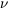/ B(Tc). The dilution factor is of importance when the source of radiation subtends only a small angle, as seen from the absorbing gas. [H76] DIMDiffuse Interstellar Medium Dimension (a) A geometrical axis. [F88] (b) An independent axis or direction in space or spacetime. The familiar space around us has three dimensions (left-right, back-forth, up-down) and the familiar spacetime has four (the previous three axes plus the past-future axis). Superstring theory requires the universe to have additional spatial dimensions. [G99] Dimensional Analysis A technique for checking the validity of a solution to a problem, a unit, or an equation in physics. It depends on the fact that, once a unit system has been defined fully (as in SI), each quantity can be expressed in terms of the base quantities in only one way. Here those base quantities are called dimensions. The table shows, first, the three dimensions used in mechanics, and, second, some other quantities in terms of these. For instance, the relationship between pressure, force, and area can be checked. Force divided by area has dimensions MLT-2/L2, i.e. dimensions ML-1T-2, as for pressure. [DC99] Dimensions Physically significant quantities usually have dimensions associated with them. The fundamental dimensions are those of mass M, length L and time T. The dimensions of other quantities can be expressed in terms of these fundamental dimensions. So, for example, momentum has dimensions of mass × velocity (MLT-1) and energy dimensions of force × distance (ML2T-2). One may define certain quantities in which the dimensions cancel out. These dimensionless quantities are significant in that they are independent of the conventions used to define units of mass, length and time. [CD99] Diode An electronic device with two electrodes that exhibits rectifying action when a potential difference is applied. Current flows for one direction of the potential (the forward direction), but when the potential is reversed the current is normally zero or very small. There are two principal types of diode: 1. The semiconductor diode consists of a single p-n junction. The current across the junction increases exponentially with voltage in the forward direction; in the reverse direction there is only a very small (leakage) current until breakdown occurs. 2. The thermionic diode consists of a heated negative cathode emitting electrons (by thermionic emission) in a vacuum with a positive anode, which accepts electrons when a positive potential is applied to it. The emitted electrons form a 'cloud' around the cathode, called the space charge. Since electrons repel each other owing to their like charges, the space charge limits the current as the voltage is increased. When the voltage is high enough the current reaches a maximum saturation value. Some other types of semiconductor diode are the light-emitting diode (LED), the tunnel diode, and the Zener diode. [DC99] Dione Fifth satellite of Saturn. Radius about 440 km. P = 4.5 days. Discovered by Cassini in 1684. [H76] Diopter (a) Symbol: D The unit of power of a lens or mirror, equal to the reciprocal of the focal distance in meters. A 5 D lens has a focal distance of 0.2 m; a diverging mirror of focal distance 1250 mm has a power of -0.8 D. Power measures the ability of a reflector, refracting surface, or lens to converge a parallel beam. The diopter is often now called the radian per meter (rad m-1). [DC99] (b) The power of a lens system is called the vergency and the diopter is the metric unit in which the power is measured. It is equal to the reciprocal of the focal length when the latter is measured in metres so that a lens with a focal length of 0.5 m has a power of two diopters. In ophthalmic work the powers of converging lenses are considered to be positive, those of diverging lenses to be negative. An alternative definition for the diopter is the vergency of a lens system having a focal length of one metre in a medium with a refractive index of unity. The diopter was adopted as a unit at a medical conference in Brussels in 1875 but the idea of defining the power of a lens as the reciprocal of its focal length was suggested seven years earlier by Nagel. [JM92] Dipole A system composed of two equal charges of opposite sign, separated by a finite distance; e.g., the nucleus and orbital electron of a hydrogen atom. An ordinary bar magnet is a dipole. [H76] Dipole Anisotropy A variation in the intensity of the cosmic background radiation caused by the motion of the Milky Way. Because the Milky Way, our galaxy, is not moving precisely with the uniform expansion of the universe as a whole, the cosmic background radiation appears slightly more intense in the direction of our peculiar motion and slightly less intense in the opposite direction. This effect, which can actually be used to measure the speed and direction of our peculiar motion, is called the dipole anisotropy. [LB90] Dipole Antenna A type of array consisting of a system of dipoles often used with radio telescopes. It differs from the dish antenna in that it consists of many separate antennas that collect energy by feeding all their weak individual signals into one common receiving set. [H76] Dipole Moment Measure of how polarized a molecule is. (How large the dipole is). [SEF01] Dirac Equation (a) The equation in quantum mechanics that describes the time evolution of the state associated with electrons and other spin * particles. Unlike Schrodinger's equation that it replaces, Dirac's equation is consistent with the special theory of relativity. [D89] (b) Mathematical description of the electron, derived by Paul Dirac, that incorporates both quantum mechanics and special relativity. [F88] (c) An equation that describes the behavior of subatomic particles called electrons. The Dirac equation incorporates both the laws of Special Relativity and the laws of Quantum Theory. (see Quantum Mechanics; Special Relativity) [LB90] Dirac Function see Delta Function. [H76] Dirac Large Number Hypothesis The current age of the universe, divided by the time it takes light to cross the radius of a proton, is about 1040. This number is also approximately equal to the ratio of the strengths of the electromagnetic and gravitational forces. Dirac felt that the approximate equality of these two large numbers was too unlikely to be accidental and that some physical process must be at work to maintain the equality. Since the first number clearly changes in time (because the age of the universe is increasing), Dirac proposed that the "fundamental constants of nature" entering the second number should also change in time, to maintain the equality. [LB90] Direct Motion (a) Motion of a solar-system body from west to east across the sky. [H76] (b) For orbital motion in the solar system, motion that is counterclockwise in the orbit as seen from the north pole of the ecliptic; for an object observed on the celestial sphere, motion that is from west to east, resulting from the relative motion of the object and the Earth. [S92] Direction Cosine A means of specifying the direction of a celestial object. The direction cosine involves the inclination of a vector to each of three mutually perpendicular axes defined through a point in space. [H76] Dirty Ice Interstellar ice grains with graphite particles or other impurities adsorbed on their surfaces. [H76] Discriminator A circuit that selects certain electrical signals and rejects others, according to a particular property of the signal, such as amplitude, frequency, or phase. For example, a pulse-height analyzer selects signals having a particular amplitude range, and a frequency discriminator picks out different frequencies. [DC99] Dish A large parabolic ``mirror" of sheet metal or wire mesh which collects radio energy and reflects it to the antenna at the focus. [H76] Disintegration The breaking up of unstable nuclei into two or more fragments, either spontaneously or as a result of bombardment by fast-moving particles. The disintegration of 7Li by proton bombardment in Cockcroft and Walton's experiment is an example of the latter type; two 4He nuclei (alpha particles) are produced. [DC99] Dissipation When a galaxy forms and gas clouds start crashing into and impeding one another. The Galaxy's disk probably formed through dissipation. [C95] Disjoint An adjective applied in mathematics to two or more sets which have no members in common. [H76] DiskThe central plane of a galaxy, as distinguished from other structural and mprphological components such as its bulge, halo or nucleus. Rotationally supported and thought to be the result of angular-momentum conserving dissipative processes that occurred during the gravitational collapse and subsequent evolution of gas-rich galaxies. Population I objects (young stars, HII regions, dust and spiral structure) are largely confined to the disk. [BFM2006] Disk Star A star located in, and orbiting with, the more general disk population (see above) of a given galaxy. [BFM2006] Disorder The departure of the atoms of a solid from their regular lattice positions. [D89] Dispersion (a) During refraction, rays can be deviated (changed in direction) by angles related to the refractive index of the medium. However, the refractive constant of any medium varies with the wavelength of the transmitted radiation. Thus, if a narrow beam of radiation of mixed wavelengths is deviated by refraction, the angle of deviation will vary with wavelength. This is called dispersion; the radiation is spread out. It can be used to produce the color spectrum of white light using a prism. It can also be a problem, causing chromatic aberration in lenses. Dispersion is actually an effect in which radiations having different wavelengths travel at different speeds in the medium. Normally the refractive index increases as the wavelength decreases, but there are regions, where the radiation is partially absorbed, in which refractive index decreases with decreasing wavelength. (see Anomalous Dispersion) [DC99] (b) The separation of a beam of light into the individual wavelengths of which it is composed by means of refraction or diffraction. [McL97] (c) Resolution of white light into its component wavelengths, either by refraction or by diffraction. [H76] (d) The selective retardation of radio waves when they propagate through an ionized gas. The speed of propagation depends on the frequency - the lower the frequency, the greater the time lag. Thus if a pulsar emits a sharp pulse of radio waves containing a spread of wavelengths, the waves will interact with the charged particles in the interstellar medium, and the higher frequencies will arrive at the Earth slightly before the lower frequencies. [H76] Dispersion Measure A term used in radio astronomy to describe the amount of dispersion in a radio signal. It is proportional to the product of the number of interstellar electrons per cm3 and the distance to the source (in parsecs). [H76] Dispersion Relations Formal relationships between the real and the imaginary parts of a complex mathematical function. In physical terms dispersion relations have been used to relate terms in the scattering matrix for elementary particle theories. [P88] Dispersive Power Symbol:A measure of the variation for a medium of refractive constant n with wavelength λ. It is given by the ratio:= (nb - nr) / (ny - 1) Here, nb, nr, and ny are absolute refractive constants for blue, red, and yellow light. More accurate values are obtained by using three monochromatic spectral lines of known wavelength: F (blue), C (red), and D (yellow). [DC99] Displacement The distance (linear or angular) of an oscillating particle from its equilibrium position at any instant. [H76] Dissipation The removal of energy from a system to overcome some form of resistive force (mechanical or electrical). Without resistance (as in motion in a vacuum and the current in a superconductor) there can be no dissipation. Dissipated energy normally appears as thermal energy. [DC99] Dissipative Structures states of matter arising through bifurcation when a system is driven away from the state of thermodynamic equilibrium by external constraints exceeding a critical value. [D89] Dissipative System a system satisfying the second law of thermodynamics. Such a system gives rise to irreversible processes, associated with a time-asymmetric evolution of observable quantities. [D89] Dissociation When a compound separates into two or more parts. [SEF01] Dissociative Recombination The capture of an electron by a positive molecular ion, after which part of the recombination energy results in the dissociation of the molecule into two neutral atoms. [BFM2006] Distance Ladder see Cosmic Distance Ladder. [HH98] Distance Modulus Difference between the apparent (m) and the absolute (M) magnitude of an astronomical objects: (m-M) = 5 log (r / 10) or -5 + 5 log(r), where r is in parsecs. It is a compact way to logarithically represent linear distances having an intrinsically large dynamic range. For instance, an object at 10 pc has a distance modulus of 5 mag; 1 kpc (= 1,000 pc) has a distance modulus of 10.0 mag, and 1 Mpc (= 1,000,000 pc) has a corresponding distance modulus of 25.0 mag. mag. [BFM2006] Distribution Function A function that gives the relative frequency with which the value of a statistical variable may be expected to lie within any specified interval (cf. statistical error). For example, the Maxwellian distribution of velocities gives the number of particles, in different velocity intervals, in a unit volume. [H76] Diurnal Motion (a) The apparent westward motion of celestial bodies, as seen from Earth, due to the Earth's axial rotation. [H76] (b) The apparent daily motion of celestial bodies across the sky from east to west, caused by the Earth's rotation. [S92]UT1 The predicted value of the difference between UT1 and UTC, transmitted in code on broadcast time signals:UT1 = UT1 - UTC. (see Universal Time; Coordinated Universal Time.) [S92] Diverging Lens A lens that can refract a parallel beam into a divergent beam. Diverging lenses used in air are thinner in the middle than at the edge. They may be biconcave, plano-concave, or concavo-convex in shape. As these lenses have negative power, they are sometimes called negative lenses. Compare converging lens. [DC99] DLA Systems Damped Lyman Alpha Systems are a subset of quasar absorption line systems classically defined as having HI (neutral hydrogen) column densities in excess of 2 x 1020 atoms/cm2. They are identified by their wide dmaped Lyman /alpha absorption profiles, and all DLA's (to date) show metal-line absorption. DLA's dominate the neutral gas content of the Universe and may be expected to constitute the primary reservoir of star-forming gas at high redshift. Note: Metal-Strong Damped Lyman Alpha Absorbers (MSDLA) constitute a small subset of the DLA class [BFM2006] DMA Direct Memory Access An efficient electronic method of transferring digital (numerical) information or data to a computer. [McL97] dKe, dMe Stars K or M dwarfs with hydrogen emission lines. [JJ95] DN Data Number Also called ADU. One count or one (least significant) bit; the smallest unit of an analog-to-digital conversion system. For example, a 10-volt 16-bit system converts the range 0-10 volts into 65,536 levels or data numbers. [McL97] DNA DeoxyriboNucleic Acid The macromolecule that carries the genetic information requisite to life on Earth. [F88] Domain a ferromagnetic material is composed of domains, in each of which there is magnetisation in a definite direction. Neighboring domains generally have opposed directions of magnetisation because that lowers the energy of the magnetic body, so that the magnetisation per unit volume of the whole body is generally very much less than that of single domains. A domain wall is the boundary between two domains. [D89] Doping The process of selectively adding known amounts of foreign atoms into the silicon (or other semiconductor) crystal to subtly change its electrical properties. If the doping results in excess electrons then the material is called n-type, if it results in an excess number of positively charged sites (holes) it is called p-type. [McL97] Doppler Broadening (a) The increase in the width of a spectral line as a result of the motion of the particles emitting or absorbing the radiation. (see Monochromatic Radiation) [DC99] (b) The broadening of spectral lines caused by the thermal, turbulent, or mass motions of atoms along the line of sight. Small displacements of radiation absorbed or emitted by these atoms toward longer and shorter wavelengths result in broadening of the lines. [H76] Doppler Effect (a) The alteration in frequency of electromagnetic radiation due to relative motion between the source and observer. [C97] (b) An apparent change in the frequency of a wave motion, caused by relative motion between the source and the observer. It is found with sound waves: as the source and observer move together the apparent frequency of the sound is higher than that produced; as they move apart it is lower. An example is that of an approaching motorbike - the pitch of the engine sound appears to drop suddenly as it passes. The apparent frequency (f') of sound as a result of relative motion between source and observer in a direct line is given by: f ' = (V + U0) f / (V - Us) where V is the speed of sound, U0 the observer's velocity, Us the source velocity, and f the source frequency. (c) A similar effect for electromagnetic radiation. The radiation emitted by a source with a motion component towards the observer will have increased frequency: if the source is moving away from the observer, the observed frequency is reduced. This has great significance in astronomy. Thus the Doppler effect on radar pulses reflected by the planets gives information on their rotation. The displacement of lines in the spectra of stars and galaxies (i.e. the red shift or the blue shift) can tell the astronomer how these bodies are moving. For electromagnetic radiation the theory of the effect is not the same as for sound. This is because there is no fixed medium to give a frame of reference. Relativity theory gives the following result: v = v0 {[(1 - v / c) / (1 + v / c)]}1/2 Here v is the observed frequency, v0 is the emitted frequency; v is the speed of separation of source and observer, related to the speed of light c. If v is small compared to c the expression simplifies to: v = v0 (1 - v / c) [DC99] (d) The apparent change of frequency or wavelength of radiation from an object due to its motion toward or away from us. If the object is receding the frequency is decreased and the wavelength is increased, i.e. becomes red-shifted. [McL97] (e) Displacement of spectral lines in the radiation received from a source due to its relative motion along the line of sight. A motion of approach results in a blueshift; a motion of recession results in a redshift. [Silk90] (f) Effect on the wavelengths of light (or sound) emitted by a source at a distance that is increasing or decreasing in relation to the observer. If the distance is increasing, the wavelengths are "stretched" (the light received shifts towards the red end of the spectrum; sound received goes down in pitch). If the distance is increasing, the wavelengths are "squeezed" (the light received shifts towards the blue end of the spectrum; sound received goes up in pitch). [A84] Doppler Shift (a) The blueshift or redshift produced by an object's motion toward or away from us. If a star moves toward us, its light waves get compressed and its spectrum is blueshifted; if a star moves away from us, its light waves get stretched and its spectrum is redshifted. The Doppler shift allows astronomers to measure the radial velocities of stars. The Doppler shift is not responsible for the redshifts that most galaxies exhibit; that is a cosmological redshift. [C95] (b) Change in the apparent wavelength of radiation (e.g., light or sound) emitted by a moving body. A star moving away from the observer will appear to be radiating light at a lower frequency than if at rest; consequently, lines in the star's spectrum will be shifted toward the red (lower frequency) end of the spectrum. The existence of a direct relationship between the redshift of light from galaxies and their distances is the fundamental evidence for the expansion of the universe. [F88] (c) Displacement of spectral lines in the radiation received from a source due to its relative motion in the line of sight. Sources approaching (-) the observer are shifted toward the blue; those receding (+), toward the red. The Doppler shift makes it possible to determine the radial velocity and the rotation of stars. [H76] S Doradus A supergiant eclipsing binary (an Eta Carinae-type object) in the Large Magellanic Cloud. It has a period of about 40 years. [H76] 30 Doradus (NGC 2070) A giant HII region, at least 300 pc across - one of the largest known - in the Large Magellanic Cloud. It is larger and more luminous than any known in the Galaxy. It is the brightest object (Mv = - 19) in the Cloud at both optical and radio wavelengths, and contains the densest concentration of W-R stars. (The brightest object near the center is a O+ WN star of Mv = - 10.2.) It is characterized by very rapid, disordered, and complex motions. [H76] Double Bond A pair of shared electrons. Atoms at either end cannot rotate around the bond. Also called pi bond or James-James. [SEF01] Double Cluster in Perseus see h and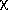Persei. [H76] Double Galaxies Two galaxies in orbit about each other; same as "binary galaxies." [LB90] Double Radio Source A radio galaxy, the bulk of whose radio emission comes from two sources on opposite sides of the visual galaxy. The radiation is presumably the result of an explosion in the nucleus of the parent galaxy, which caused the ejection at high speed of energetic particles in two opposite directions. About one-third of all known radio galaxies are double sources. [H76] Double Star (a) A ``system" of two stars that appear - because of coincidental alignment when viewed from Earth - to be close together; it is, however, an optical effect only, and therefore not the same as a binary star system (although until the twentieth century there were few means of distinguishing double and binary stars). [A84] (b) A star system having two stars that revolve around each other. [C95] Doublet (a) A compound lens with two elements, often in contact. The purpose is usually to reduce aberrations. If the lenses are in contact, the power of the doublet is the sum of the powers of the elements. Thus, P = P1 + P2 or, 1/f = 1/f1 + 1/f2 (f is focal distance). A 5 D lens combined with a -2.5 D lens forms a doublet of power 2.5 D, or focal distance 400 mm. [DC99] (b) In a spectrum, a pair of associated lines arising from transitions having a common lower energy level. [H76] DQE Detective Quantum Efficiency The ratio of the actual number of detected photoelectrons in a detector to the number of incident photons when proper account is taken of noise and other efficiency factors. [McL97] Diameter Distance Any distance to a celestial object which is based upon the use of a standard ruler. [C97] Down A flavor of quark. (see Flavor) [G97] Down-Loading The process of passing data or a program from one computer to another, often in a hierarchical structure where the source computer is the more powerful one. [McL97] Draco 1. A constellation in the northern sky. 2. A dwarf galaxy that orbits the Milky Way and lies about 250,000 light-years from the Galactic center. Draco is the least luminous galaxy known, with an absolute magnitude of -8.6. [C95] Draco System A dwarf elliptical galaxy, about 80 kpc distant, the intrinsically faintest (Mv = - 8.5) member of the Local Group (next to And I-III). Diameter about 1 kpc. [H76]Dra see Thuban. [H76] Drell-Yan Annihilation annihilation of a quark and an antiquark, thereby producing a high energy or high momentum photon, W or Z boson. [D89] Draconic Month see Nodical Month. [H76] Drift Curves In radio astronomy, the output response as a function of position for a given filter as the source passes through the beam (cf. velocity profile). [H76] DRAGN Double Radio Source Associated with a Galactic Nucleus Large-scale double radio sources produced by outflows (jets) that are launched by processes in active galactic nuclei. Term coined by P. Leahy in 1993 (in Jets in Extragalactic Radio Sources, eds. Röser, H.-J., & Meisenheimer, K.; Berlin: Springer-Verlag). DRO Direct Read-Out [LLM96] Dry Ice Solid Carbon Dioxide (CO2). An inexpensive cryogen for cooling detectors. [McL97] DSB Double SideBand [LLM96] DSP Digital Signal Processor A special kind of computer chip. [McL97] Dual, Duality, Duality Symmetries Situation in which two or more theories appear to be completely different, yet actually give rise to identical physical consequences. [G99] Duality Wave-particle Duality: the concept that some entities show both wave and particle properties. There are two cases. (a) Electromagnetic Radiation. Einstein (1905) argued that, whereas the propagation of these radiations is fully described by the theory of electromagnetic waves, interaction with matter occurs as if the radiation travels in packets (photons) each with a quantum of energy hv, where h is the Planck constant and v is the frequency of the wave. His examples were the Planck radiation law, photoelectric emission, and photoionization of gases. The principle has been verified for radiations of all wavelengths from microwaves to gamma rays, in many different phenomena involving emission, absorption or scattering. Whereas such wave properties as frequency, phase speed, and electric and magnetic fields are measurable quantities, photons are only observable when being created or destroyed. (b) Particle Waves. In 1924 de Broglie suggested that atomic particles might have wave-like properties and that the wavelength (λ) would have the same relationship to the momentum (p) as applies to electromagnetic radiation: λ = h / p. This concept has been used very successfully in the form of quantum theory called wave mechanics. Beams of particles including electrons, neutrons, and gas molecules incident on crystals form diffraction patterns analogous to those given by x-rays. The wavelength formula is fully verified. [DC99] Dual Resonance Theory A theoretical model that was developed to explain some of the experimental properties of the scattering matrix, such as the duality between s- and t-channel scattering. It was Veneziano's version of the dual resonance model that paved the way for Nambu's string theory. [P88] Dubnium A radioactive synthetic element made by bombarding 249Cf nuclei with 15N atoms. It was first reported by workers at Dubna, a town near Moscow. Symbol: Db; p.n. 105; most stable isotope 262Db (half-life 34 s). [DC99] DUMAND Deep Underwater Muon and Neutrino Detector [LLM96] Dumbbell Galaxies (db) According to Matthews, Morgan and Schmidt (1964, Ap.J., 140, 35) Dumbbell (db) Galaxies are a group of objects allied to the D ("dustless") galaxies, in which two, separated but approximately equal-sized nuclei are observed in a common envelope. They may well be related to galaxies that have one or more fainter components in their envelopes. Dumbbell galaxies are then the extreme cases of very close multiple galaxies in which there are only two, but equal, components. A catalog of dumbbell galaxies can be found in Valentijn and Casertano (1988, Astron. Astrophys., 206, 27.) [H76] Dumbbell Nebula A planetary nebula of large apparent diameter and low surface brightness in Vulpecula about 220 pc distant. (M27, NGC 6853) [H76] Duty Cycle The fraction of time a pulsed beam is on. [H76] DVR Direct Voltage Readout Dwarf (a) Luminosity Class V stars, essentially defining the Main Sequence, that are currently supported by the fusion of hydrogen into helium in their cores. (b) A small, faint galaxy, exemplified locally by such systems as Ursa Minor, Draco, Sculptor, Sextans, Carina, Fornax, Leo II, and Leo I, and the Andromeda dwarfs orbiting M31. Dwarf Cepheids Type I Cepheids (Spectral Class A-F) ( +4 to +2) with periods of 1 to 3 hours. (also calledScuti stars) [H76] Dwarf Galaxy A galaxy at the faint end of the general lumisosity function and generally exhibiting ing low surface brightness. [BFM2006] Dwarf Nova (a) A short-period binary system consisting of a hot white dwarf (or a hot blue sdBe subdwarf) and a much cooler and slightly more massive late-type main-sequence companion which fills its Roche lobe and is ejecting mass onto the white dwarf through its inner Lagrangian point. (The light from dwarf novae comes from four sources: a white dwarf, a cool main-sequence star, a hot spot, and a disk.) The outbursts are usually assumed to be caused by the explosive nuclear burning of hydrogen-rich material accreted onto the surface of a degenerate star. (see also SS Cyg star, U Gem star) [H76] (b) Cataclysmic variables in which the brightness increases suddenly at intervals ranging from several days to years. [JJ95] Dwarf Stars Main-sequence stars with masses equal to or less than that of the Sun. More generally, any star on or below the Main Sequence in the Hertzprung-Russell diagram. [F88] Dyad An operator indicated by writing the symbols of two vectors without a dot or cross between them. [H76] Dye Laser A widely used laser which has a solution of dye molecules as its active element, excited by photon pumping. The dye can emit over a wide range of frequencies making it an ideal source of radiation tunable across the whole visible spectrum. Its large bandwidth makes it a good source of short optical pulses. [D89] Dynamic Range (a) The ratio of the maximum possible signal (or saturation level) to the system noise floor. [McL97] (b) The maximum variation in signal input, over which the detector output represents the photon flux, without losing significant amounts of information. [R04] Dynamical Equinox The ascending node of the Earth's mean orbit on the Earth's equator; i.e., the intersection of the ecliptic with the celestial equator at which the Sun's declination is changing from south to north. (see Catalog Equinox; Equinox.) [S92] Dynamical Friction (a) The process by which a large mass gets slowed down as it moves through a sea of smaller objects and feels their gravitational pull. For example, a satellite galaxy that moves through the Milky Way's dark halo gets slowed down by the dynamical friction with the dark matter and will spiral into the Milky Way as a result. [C95] (b) That effect of stellar encounters which damps the initial motion of a star in the direction of its motion, in contrast to the diffusion process which randomizes it. [H76] Dynamical Method A method of measuring the mass of a galaxy, cluster, or even the universe which makes use of the gravitational interactions of two or more bodies. [HH98] Dynamical Parallax The "parallax" (i.e., distance) for a binary star whose orbit is well known, derived by using the mass-luminosity relation and Newton's generalization of Kepler's third law. [H76] Dynamical Time The family of timescales introduced in 1984 to replace ephemeris time as the independent argument of dynamical theories and ephemerides. (see Barycentric Dynamical Time; Terrestrial Dynamical Time.) [S92] Dynamics (a) The study of the motion and equilibrium of systems under the influence of force(s). [F88] (b) The physics that explains how particles and systems move under the influence of forces. The dynamical laws of a theory give a quantitative statement of the response of a particle to an applied force. [LB90] Dynamo An electric generator that employs a spinning magnetic field to produce electricity. [F88] Dyne The cgs unit of force necessary to impart an acceleration of 1 cm sec-2 to a mass of 1 gram. [H76] Dynode Intermediate surface within a photomultiplier tube which emits multiple electrons when bombarded by a single electron. [McL97] Dysprosium A soft malleable silvery element of the lanthanoid series of metals. It occurs in association with other lanthanoids. One of its few uses is as a neutron absorber in nuclear reactors; it is also a constituent of certain magnetic alloys. Symbol: Dy; m.p. 1412°C; b.p. 22562°C; r.d. 8.55 (20°C); p.n. 66; r.a.m. 162.50. [DC99]